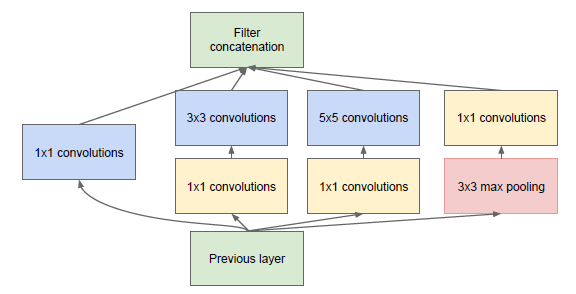## 前言

• 都提出了基础块的思想
• 均是为了克服网络逐渐变深带来的问题

• 参数数量增加
• 梯度消失和梯度爆炸
• 计算复杂度增加

• 输出层不同
• 克服网络加深弊端的方式不同

VGG是在LeNet、AlexNet的基础上引入了基础块的思想，但是在网络架构、输出等放并没有进行太多的改变，在输出层方面同样是采用连续三个全连接层，全连接层的输入是前面卷积层的输出经过reshape得到。

Inception块

• 简化版
• 降维版

• AlexNet采用5个卷积层
• VGG把5个卷积层替换成5个卷积块

## 编程实践

• Inception块
• 卷积层
• 池化层
• 线性层

Inception块

``````def inception_block(X, c1, c2, c3, c4, name):
in_channels = int(X.get_shape()[-1])
# 线路1
with tf.variable_scope('conv1X1_{}'.format(name)) as scope:
weight = tf.get_variable("weight", [1, 1, in_channels, c1])
bias = tf.get_variable("bias", [c1])
p1_1 = tf.nn.conv2d(X, weight, strides=[1, 1, 1, 1], padding="SAME")
​
# 线路2
with tf.variable_scope('conv2X1_{}'.format(name)) as scope:
weight = tf.get_variable("weight", [1, 1, in_channels, c2])
bias = tf.get_variable("bias", [c2])
p2_1 = tf.nn.conv2d(X, weight, strides=[1, 1, 1, 1], padding="SAME")
p2_shape = int(p2_1.get_shape()[-1])
with tf.variable_scope('conv2X2_{}'.format(name)) as scope:
weight = tf.get_variable("weight", [3, 3, p2_shape, c2])
bias = tf.get_variable("bias", [c2])
p2_2 = tf.nn.conv2d(p2_1, weight, strides=[1, 1, 1, 1], padding="SAME")

``````def conv_layer(self, X, ksize, out_filters, stride, name):
in_filters = int(X.get_shape()[-1])
with tf.variable_scope(name) as scope:
weight = tf.get_variable("weight", [ksize, ksize, in_filters, out_filters])
bias = tf.get_variable("bias", [out_filters])
conv = tf.nn.conv2d(X, weight, strides=[1, stride, stride, 1], padding="SAME")
return activation
​
def pool_layer(self, X, ksize, stride):
return tf.nn.max_pool(X, ksize=[1, ksize, ksize, 1], strides=[1, stride, stride, 1], padding="SAME")``````

``````def linear(self, X, out_filters, name):
in_filters = X.get_shape()[-1]
with tf.variable_scope(name) as scope:
w_fc = tf.get_variable("weight", shape=[in_filters, out_filters])
b_fc = tf.get_variable("bias", shape=[out_filters], trainable=True)
fc = tf.nn.xw_plus_b(X, w_fc, b_fc)
return tf.nn.relu(fc)``````

``````def create(self, X):
# 模块1
module1_1 = self.conv_layer(X, 7, 64, 2, "module1_1")
pool_layer1 = self.pool_layer(module1_1, 3, 2)
​
# 模块2
module2_1 = self.conv_layer(pool_layer1, 1, 64, 1, "modul2_1")
module2_2 = self.conv_layer(module2_1, 3, 192, 1, "module2_2")
pool_layer2 = self.pool_layer(module2_2, 3, 2)
​
# 模块3
module3a = self.inception_block(pool_layer2, 64, (96, 128), (16, 32), 32, "3a")
module3b = self.inception_block(module3a, 128, (128, 192), (32, 96), 64, "3b")
pool_layer3 = self.pool_layer(module3b, 3, 2)
​
# 模块4
module4a = self.inception_block(pool_layer3, 192, (96, 208), (16, 48), 64, "4a")
module4b = self.inception_block(module4a, 160, (112, 224), (24, 64), 64, "4b")
module4c = self.inception_block(module4b, 128, (128, 256), (24, 64), 64, "4c")
module4d = self.inception_block(module4c, 112, (144, 288), (32, 64), 64, "4d")
module4e = self.inception_block(module4d, 256, (160, 320), (32, 128), 128, "4e")
pool_layer4 = self.pool_layer(module4e, 3, 2)
​
# 模块5
module5a = self.inception_block(pool_layer4, 256, (160, 320), (32, 128), 128, "5a")
module5b = self.inception_block(module5a, 384, (192, 384), (48, 128), 128, "5b")
​
pool_layer5 = tf.nn.avg_pool(module5b, ksize=[1, 7, 7, 1], strides=[1, 1, 1, 1], padding="VALID")
flatten = tf.reshape(pool_layer5, [-1, 1024])
dropout = tf.nn.dropout(flatten, keep_prob=self.keep_prob)
linear = self.linear(dropout, self.num_classes, 'linear')
return tf.nn.softmax(linear)``````

``````def main():
X = np.random.normal(size=(5, 224, 224, 3))
images = tf.placeholder("float", [5, 224, 224, 3])
writer = tf.summary.FileWriter("logs")
with tf.Session() as sess:
sess.run(tf.global_variables_initializer())
prob = sess.run(model, feed_dict={images: X})
print(sess.run(tf.argmax(prob, 1)))

# 输出
module1_1:      (5, 112, 112, 64)
pool_layer1:    (5, 56, 56, 64)
module2_1:      (5, 56, 56, 64)
module2_2:      (5, 56, 56, 192)
pool_layer2:    (5, 28, 28, 192)
module3a:       (5, 28, 28, 256)
module3b:       (5, 28, 28, 480)
pool_layer3:    (5, 14, 14, 480)
module4a:       (5, 14, 14, 512)
module4b:       (5, 14, 14, 512)
module4c:       (5, 14, 14, 512)
module4d:       (5, 14, 14, 528)
module4e:       (5, 14, 14, 832)
pool_layer4:    (5, 7, 7, 832)
module5a:       (5, 7, 7, 832)
module5b:       (5, 7, 7, 1024)
pool_layer5:    (5, 1, 1, 1024)
flatten:        (5, 1024)
linear:         (5, 1000)``````

## 链接

github.com/Jackpopc/aiL

Going Deeper with Convolutions

arxiv.org/pdf/1409.4842

Network In Network

arxiv.org/pdf/1312.4400

## 作品精选

Jackpop：目录 | 精选CV、Python等系列教程

Jackpop：大数据处理 | Spark&HDFS集群配置及基本使用

Jackpop：实用工具 | 推荐3款令人惊艳的截图工具

Jackpop：强烈推荐 | 这将会成为一个优质的github项目

Jackpop：计算机小白如何开始机器学习的学习，有入门课程推荐吗?

Jackpop：C盘快满了，该如何清理？

Jackpop：2019 年双十一有哪些值得购买的东西？

more_vert